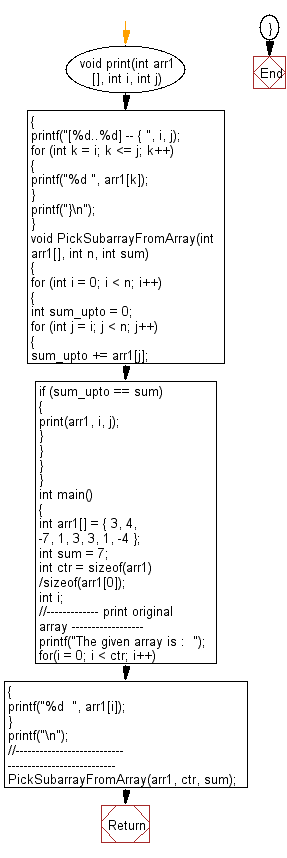﻿ C exercises: Find a subarray with given sum from the given array - w3resource# C Exercises: Find a subarray with given sum from the given array

## C Array: Exercise-47 with Solution

Write a program in C to find a subarray with given sum from the given array.

Sample Solution:

C Code:

``````#include <stdio.h>

void print(int arr1 [], int i, int j)
{
printf("[%d..%d] -- { ", i, j);
for (int k = i; k <= j; k++)
{
printf("%d ", arr1[k]);
}
printf("}\n");
}

void PickSubarrayFromArray(int arr1[], int n, int sum)
{
for (int i = 0; i < n; i++)
{
int sum_upto = 0;
for (int j = i; j < n; j++)
{
sum_upto += arr1[j];
if (sum_upto == sum)
{
print(arr1, i, j);
}
}
}
}

int main()
{
int arr1[] = { 3, 4, -7, 1, 3, 3, 1, -4 };
int sum = 7;
int ctr = sizeof(arr1)/sizeof(arr1);
int i;
//------------- print original array ------------------
printf("The given array is :  ");
for(i = 0; i < ctr; i++)
{
printf("%d  ", arr1[i]);
}
printf("\n");
//------------------------------------------------------

PickSubarrayFromArray(arr1, ctr, sum);
return 0;
}
```
```

Sample Output:

```The given array is :  3  4  -7  1  3  3  1  -4
[0..1] -- { 3 4 }
[0..5] -- { 3 4 -7 1 3 3 }
[3..5] -- { 1 3 3 }
[4..6] -- { 3 3 1 }
```

Flowchart:C Programming Code Editor:

Improve this sample solution and post your code through Disqus.

What is the difficulty level of this exercise?

﻿